# Manganese Depletion and Recycling

Manganes is used to make stainless steel and aluminum alloys. The graph below shows the manganese extraction data for the world and two Verhulst function fits to the data in order to extrapolate into the future.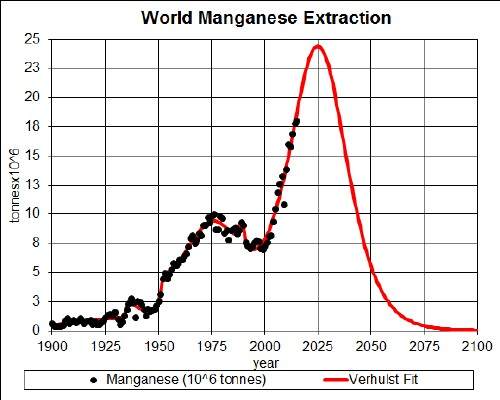Manganese extraction rate for the world and two Verhulst function fits to the data.

The blue curve is obtained by restricting the total extraction to the amount already extracted plus the estimated reserve of 500x106 tonnes; the red curve is obtained by restricting the total extraction to the amount already extracted plus the estimated reserve base of 5.2x109 tonnes.

It appears that the recent rapid rise in extraction rate is unsustainable for more that a few decades or so from now.

Taking an average extraction curve of the two fits, the crossover point at year ~2016 when the amount extracted is equal to the amount left to be extracted is shown here: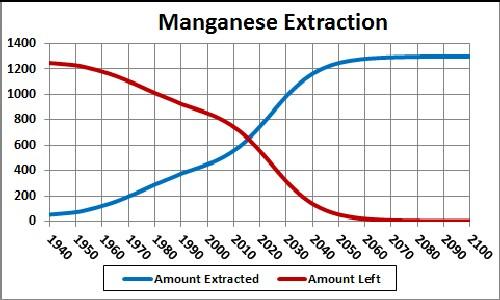## Recycling

Assume that:

• The manganese extraction curve is the average of the two curves given above.
• Recycling of manganese follows a hyperbolic tangent curve from 10% to 60% recycling with a break point of year 1990 and width 50 years, beginning in year 1970.
• The recycling is delayed by a Gaussian curve peaking at a delay of 25 years and a width of 10 years.

The effective manganese available for making items after the first ten recycling cycles is shown in the following graph, along with the effective manganese available for each cycle: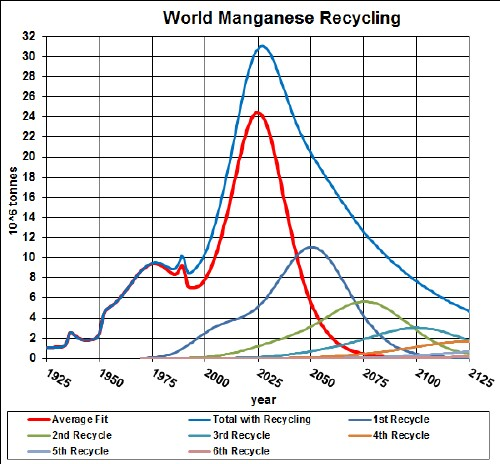The equation for a recyling cycle is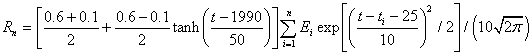,

where Ei is the amount available from the previous cycle. Here is an example of the Excel coding:

{=((\$J\$2+\$I\$2)/2+((\$J\$2-\$I\$2)/2)*TANH((A27-\$K\$2)/\$L\$2))*SUM(\$I\$27:I27*(EXP(-1*((A27-\$A\$27:A27-\$N\$2)/\$O\$2)^2/2))/\$O\$2/SQRT(2*PI()))} (The curly bracket surrounding the term makes it into an array; it must be entered by holding down the SHIFT & CTRL keys while pressing the ENTER key.)

Thus, under the assumptions given above, the effective amount of manganese available for making items peaks at about year 2050 and falls off rapidly after that. Humans will have taken concentrated manganese deposits and scattered them across the surface of the earth.

The Excel spreadsheet is set up to make it easy to calculate with different recycling assumptions.

## Manganese Per Capita

Dividing the manganese recycling curve above by the projected world population (asymptote ~1010), one gets: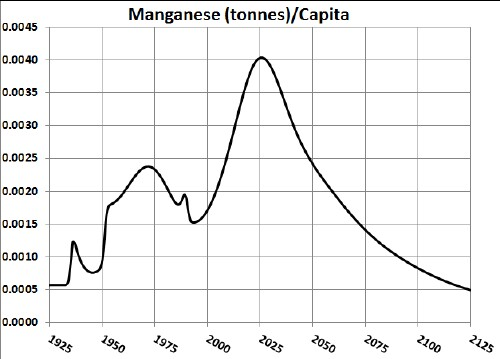Minerals Depletion